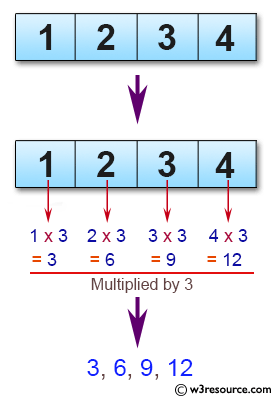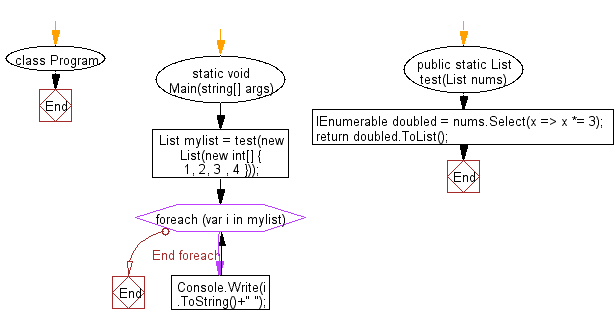﻿ C# - From a list of integers, multiply each element by 3# C# Sharp Basic Algorithm Exercises: Create a new list from a given list of integers where each element is multiplied by 3

## C# Sharp Basic Algorithm: Exercise-141 with Solution

Write a C# Sharp program to create a new list from a given list of integers where each element is multiplied by 3.

Pictorial Presentation:Sample Solution:

C# Sharp Code:

``````using System;
using System.Collections.Generic;
using System.Linq;

namespace exercises
{
class Program
{
static void Main(string[] args)
{
List<int> mylist = test(new List<int>(new int[] { 1, 2, 3 , 4 }));
foreach(var i in mylist)
{
Console.Write(i.ToString()+" ");
}
}
public static List<int> test(List<int> nums)
{
IEnumerable<int> doubled = nums.Select(x => x *= 3);
return doubled.ToList<int>();
}
}
}
```
```

Sample Output:

`3 6 9 12`

Flowchart:C# Sharp Code Editor:

Improve this sample solution and post your code through Disqus

What is the difficulty level of this exercise?

Test your Programming skills with w3resource's quiz.

﻿